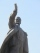The volume 2

The volume of a cube is 27 cubic meters. Find the height of the cube.

Result

h =  3 m

Solution:Leave us a comment of example and its solution (i.e. if it is still somewhat unclear...):Be the first to comment!To solve this example are needed these knowledge from mathematics:

Tip: Our volume units converter will help you with converion of volume units.

Next similar examples:

1. Cube into cylinderIf we dip a wooden cube into a barrel with a 40cm radius, the water will rise 10 cm. What is the size of the cube edge?
2. Cube in a sphereThe cube is inscribed in a sphere with volume 6116 cm3. Determine the length of the edges of a cube.
3. CubesOne cube is inscribed sphere and the other one described. Calculate difference of volumes of cubes, if the difference of surfaces in 257 mm2.
4. Pyramid heightFind the volume of a regular triangular pyramid with edge length a = 12cm and pyramid height h = 20cm.
5. Rectangular cuboidThe rectangular cuboid has a surface area 5334 cm2, its dimensions are in the ratio 2:4:5. Find the volume of this rectangular cuboid.
6. Sphere VSFind the surface and volume of a sphere that has a radius of 2 dm.
7. Axial sectionAxial section of the cone is an equilateral triangle with area 208 dm2. Calculate the volume of the cone.
8. SphereThe surface of the sphere is 2820 cm2, and weight is 71 kg. What is its density?
9. Metal tubeCalculate the metal tube mass 8dm long with the outer radius 5cm and the inner radius 4.5cm and 1cm3 of this metal is 9.5g.
10. PoolIf water flows into the pool by two inlets, fill the whole for 18 hours. The first inlet filled pool 6 hour longer than second. How long pool take to fill with two inlets separately?
11. WinchThe steel rope has a diameter of 6mm and a length of 20m. We are winding on drum width 60mm, starting diameter 50mm. What is the final diameter after winding?
12. PoolThe swimming pool is 10 m wide and 8 m long and 153 cm deep. How many hectoliters of water is in it, if the water is 30 cm below its upper edge?
13. CylindersArea of the side of two cylinders is same rectangle of 50 cm × 11 cm. Which cylinder has a larger volume and by how much?
14. TanksFire tank has cuboid shape with a rectangular floor measuring 13.7 m × 9.8 m. Water depth is 2.4 m. Water was pumped from the tank into barrels with a capacity of 2.7 hl. How many barrels were used, if the water level in the tank fallen 5 cm? Wr
15. A sculptorA sculptor is duplicating a statue based on an original statue. If the scale factor of the replication is 3.2, will the new statue be larger or smaller than the original statue (enter 1 = larger, 0= smaller)
16. IcebergWhat is the surface area of 50 cm iceberg (in the shape of a cuboid) that can carry a man with luggage with a total weight of 120 kg?
17. Prism 4 sidesThe prism has a square base with a side length of 3 cm. The diagonal of the sidewall of the prism/BG/is 5 cm. Calculate the surface of this prism in cm square and the volume in liters